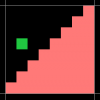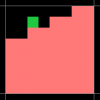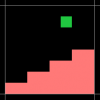# GMS 2.3+Calculate if a point inside a tile is in a certain area (slopes sorta)

#### RocketMonster

##### Member
I am trying to find an elegant way to calculate if a given point is inside a slope (of varying degrees).

A 45 is easy with the below formulae to check if the green point is inside the slope (pink);if x + y > tile_width then inside = true

Is there a math trick for checking non 45 degree slops such as...I could store the "inside" points in an array and check that but my tiles are 24x24 in game and I have over 20 non 45 degree sloped tiles, so that's a lot of numbers and a lot of work if I decide later to use smaller or larger tiles or add more tiles etc.

#### Attachments

• 1.3 KB Views: 0

#### chamaeleon

##### Member
I am trying to find an elegant way to calculate if a given point is inside a slope (of varying degrees).

A 45 is easy with the below formulae to check if the green point is inside the slope (pink);

View attachment 35981
if x + y > tile_width then inside = true

Is there a math trick for checking non 45 degree slops such as...

View attachment 35982 View attachment 35984

I could store the "inside" points in an array and check that but my tiles are 24x24 in game and I have over 20 non 45 degree sloped tiles, so that's a lot of numbers and a lot of work if I decide later to use smaller or larger tiles or add more tiles etc.
Could be encoded in binary probably in less than 2KB for 20 tiles (24*24*20/8 bytes worth of bits).

#### TheouAegis

##### Member
In your pic, x+y needs to be greater than width-2. If x is 0 and y is 7, you are inside the ground.

A slope is y=mx+b, so for each tile you would need to store its rate of climb (m) and its vertical offset (b). Then on collision checking, you would fetch each of those saved values, feed them and your x coordinate into the formula, and see if y is less than the result to know if you are in the ground.

Or you could store the pixel values in 4-byte sets. \$01234567 is your first pic, \$00112233 is your second pic.
var n = value >> (x & 7) & 15;
If y & 7 >= n
{y = (y & ~7) | n - 1;}

Last edited:

#### TheouAegis

##### Member
Now you're just counting and pretending it's hex =P
That was the example he gave me to work with!

But I reread the post and tiles are 24x24, so he'd have to have a 3x3 matrix of values per tile. At that point, mx+b would probably be the way to go as long as the tiles are simple linear slopes.

•RefresherTowel

#### RocketMonster

##### Member
Thanks everyone for taking the time to respond, looks like y=mx+b will do the trick for my needs, the byte sets are also a great idea, never crossed my mind to do that.